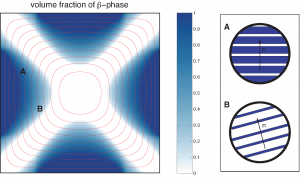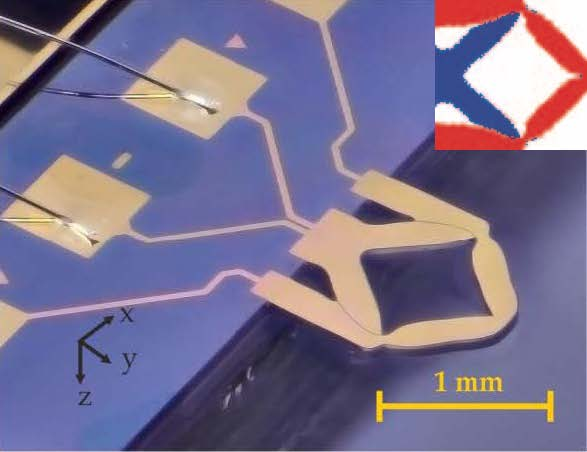Variational methods

Variational methods

Calculus of Variations, optimal design and optimal control problems and variational methods for PDE.

Calculus of Variations has been central in the research of the group since its beginning, particularly in the study of vector variational problems and in the development of new techniques applied in different scenarios of Solid Mechanics and Mechanical Engineering.The microstructure issue is included here. This comes as a generalized solution of an ill-posed variational principle, which does not admit minimizers. From a mathematical point of view, the study of relaxation for variational problems, including the central concept of quasiconvexity, is in the core of this. From a more applied side, deepen in relaxation and the mathematical theory of microstructure bring about a better insight of several physical phenomena and engineering practical questions, such as composite materials and phase transitions.

In our research, we address the two purely mathematical problems mentioned above, from both analytical and numerical views, as well as the application of variational techniques to real-world situations from a very broad perspective.

Posted in Uncategorized

Topology optimization

Topology optimization

Topology optimization is considered nowadays as a very consolidated conceptual tool for structural design, not only for pure academic purposes but also for industrial applications.

It solves the basic engineering problem of distributing a limited amount of material in a design space to optimize a certain objective function while some constraints are fulfilled.At present, to do this in an optimal way is an engineering must. Topology optimization brings together Mathematics, Physics and Engineers in this fascinating and challenging task, which is today present in multiple physical contexts. From a mathematical side, there are many deep questions relevant to the optimal design of structures, as typical optimal solutions of these problems are of microstructural nature, and therefore relaxation via variational techniques and mathematical description of microstructure are of major importance. From a more practical point of view, to device numerical algorithms capable to capture optimal microstructural solutions, or contrary to this, to find classical, black and white (and therefore manufacturable), pseudo-optimal structures are two of the main current interests in this research area.

Our focus is now on the topology optimization of hyperelastic structures (those exhibiting large deformations and displacements) and the development of the topology optimization software Toptimiz3D.

Posted in Uncategorized

Nonlocal problems

Nonlocal problems

A great variety of phenomena in Mathematical Physics and Engineering have an intrinsic nonlocal nature. In the last decade, there has been a growing interest in taking nonlocal nature into consideration in mathematical models, leading to the mathematical analysis of wide variety of nonlocal and fractional models.

We are particularly interested in nonlocal variational problems, considering questions from existence conditions to the study of relaxation when problems lack optimal solutions. From a more applied point of view, our main interest is to include nonlocal modeling into the mathematical theory of hyperelasticity, with the motivation of including typical singular situations when materials deform a lot as fracture, cavitation of multiscale phenomena in general.

Posted in Uncategorized

Optimal design of piezoelectric transducers

Optimal design of piezoelectric transducers

Piezoelectric devices have become of common use in many engineering applications nowadays, being its design and optimization a very challenging matter.

Piezoelectric materials are those having the ability to convert electrical energy into mechanical one, and vice versa. Often surface bonded to structures, they are commonly used for sensing, acting and even for reducing noise and structural vibrations as part of active control systems. The word transducer comprises both sensor and actuator terms. To get the desired effect, tailor-made electrodes are shaped in a proper way, and here is where topology optimization makes the difference. More specifically, the use of this technique permits to design transducers with promising applications at the micro scale that may go from providing therapeutic stimulations through the skin as bio-patches to sensing very fine characteristics as bio-sensors.The group has a long experience in this area, especially in the optimal design of modal transducers (those that isolate a particular mode of a structure while remaining insensitive to the non-targeted modes), and our actual challenge is the design under hyperelasticity laws, as required for the modern piezoelectric polymers.